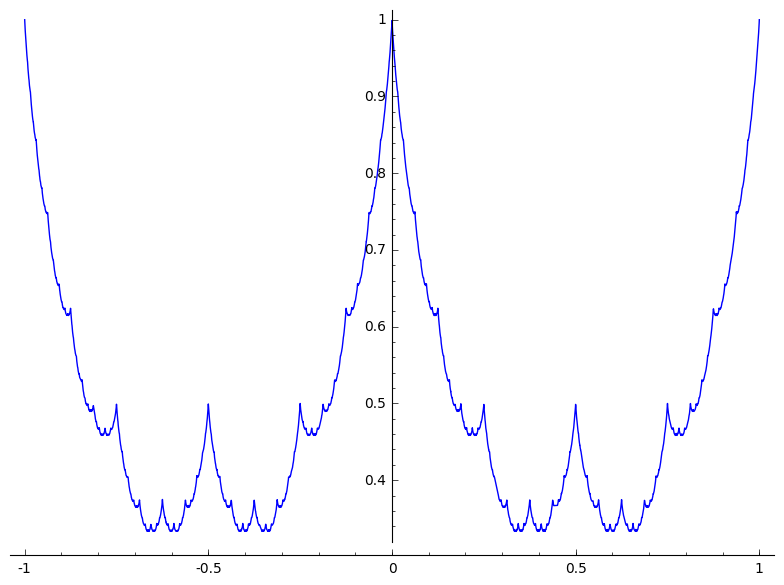# How to plot a complicated function in Sage Math

I apologize in advance for my lack of knowledge and understanding of sage. In principle what I want to achieve is the visualization of this function:

$$g \mapsto \left|x - \lfloor{x}\rfloor - \frac{1}{2} \right|$$ $$f(x) := \sum_{j=0}^\infty 2^{-j}g(2^jx)$$

Though I am having trouble finding out how to do this. In the documentation I did not find any easy way to handle infinite series.

I am sure this would be very helpful to many that struggle with a math problem and need to see it to believe it. A conventional plotting program will not work here, hence I searched for a better tool and found Sage Math. Is it possible in sage? Do you have any TLDR material that I could look into?

edit retag close merge delete

So do you want to plot a function which is 1 for x>=0 and +Infinity for x<0?

Sort by » oldest newest most voted

You can proceed as follows, where $N$ is given to serve as an approximation of $\infty$.

sage: g(x) = abs(x - floor(x) - 1/2)
sage: def f(x, N): return sum(g(2^j*x)/2^j for j in range(N+1))
sage: N = 100 # for instance...
sage: plot(f(x, N), x, -1, 1)Note. For this approach to make sense, you need to show that that the partial sums converge fast, which is the case since $g(x) \le 1/2$ for all $x$.

more